## Sunday, November 25, 2012

### f(x) = x * random ^ random

Earlier this month, I received a request from Jason Foose. These are six graphs of y = x * rand^rand: two with the TI-84+, two with the Casio Prizm (fx-CG 10, and two with the HP 39gii.

On the Prizm, I had to use the catalog to find the random number function (labeled Ran#).

Re-editing the function will cause the function to be redrawn.

Enjoy!

Eddie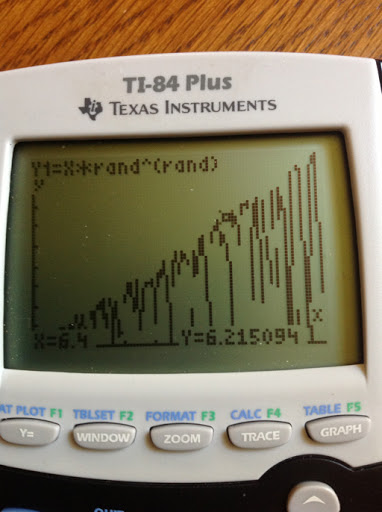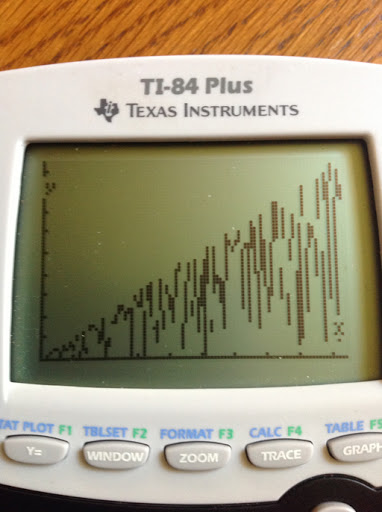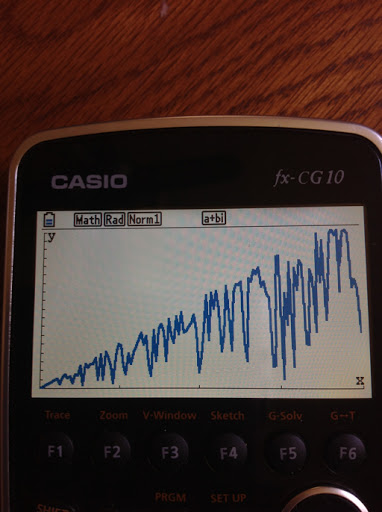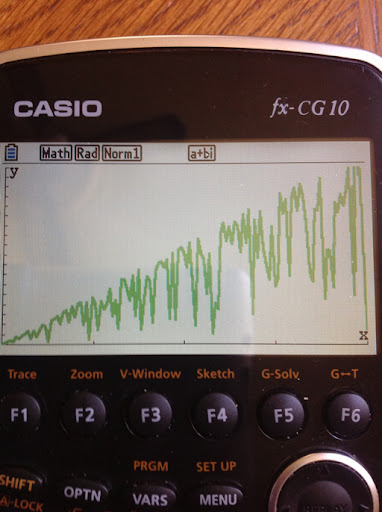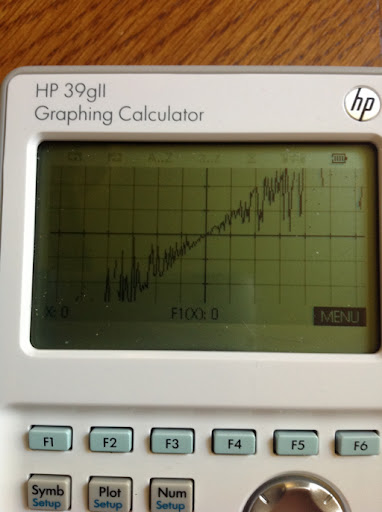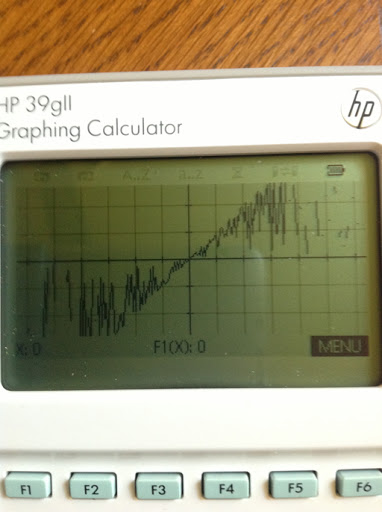1.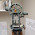That is VERY COOL!!!!! Thanks for the thought and effort Eddie. I am impressed by the screen resolution of the Casio GC-10 and the
HP 39II. Hhmmmm, what if we throw in a powerball and use the reciprocal value of Hp's normal random (<1 >0) generated value?

2.Okay. I've had some time to digest this. Why is the model still relatively predictable? There's unpredictable spikes but the there is still a smooth overlying upward trend. My initial thoughts were that this would graph maniacally. Any thoughts or insight???

3.Jason,

In a typical random number function, the number returned, let's say r, is between 0 and 1. Typically, 0 ≤ r < 1.

For r^r, we have 0^r ≤ r^r < 1^r

The lower bound is either undefined, in the case of 0^0. If r ≠ 0, 0^r = 0.

The upper bound is 1, for any r.

Now we multiply 0^r ≤ r^r < 1^r by x to get the following bounds:

0^r * x ≤ x * r^r < x

r^r is a "pseudo-coefficient" of x. While we are seeing a seemingly random pattern, the growth is expected to be linear.

Eddie

4.Hello Eddie,

I think of the "quality" behind the random. The average of random must be near of 0.5 and the standard deviation 1/(sqrt(12)).

On my hp35s, with 1000 rand and a seed of 0.5, found 0.4967 and 0,2883 (good in 1:30 minute)
HP 15c : 0,5149 and 0,2891 (average a little high, in 17 minutes and the same seed)

With r^r : i think the average near 1/sqrt(2) but have no idea for the standard deviation.

Then f(x) = x * r ^ r is in average near x/sqrt(2).

What do you think about that ?
It's time to sleep here, good night !

1.Caloubugs,

You are on track with the average.
-------------------------------
To get an idea for the sample standard deviation, I am going to generate nine lists, three each on a TI-84, HP calculator, and Excel, of 100 data points of random^random. This is no where near a proof of course, just to get an idea of what the deviation would be.

Sample Standard Deviation for each list were:
(TI)
0.2547639802
0.2575450285
0.2551388739
(HP)
0.262557143565
0.266684230048
0.265612924289
(Excel)
0.269713444
0.254391699
0.241800699

Average of the sample standard deviation from the nine lists is approximately 0.25868978, not too far away from 1/sqrt(15).

Eddie

5.Thanks Eddie !

Including random in fonctions was a discover for me and behind that, i think there's a mathematic theory (High numbers laws or something like that).

My maths studies are 20 years ago... English too :-D

It wakes my brain !

Pascal

6.OK, thinking of f(x,y)=x^y doesn't let me sleep.

Then as the average of f(x)=1 is 0.5 (intégration of f(x) between 0 and 1), the average of f(x,y)=x^y is the double intégration of f(x,y) dx dy (not really easy to write math here ;-)
We find ln 2 = 0,69315 which is correct with average of random^random.
I find the result with wiris.net

The standard deviation is then :
Sqrt( intg[0,1] intg[0,1] x^(2y) dx dy - (ln 2)^2 ) = 0,2624
Correct with your random^random standard deviation.
Calculate with www.wiris.net too...

Have fun !

Pascal

1.I was in the range with the nine list samples. Thank you posting the integration - and yes it is not easy to type math - especially using only text.

I use my iPad to type my blogs and customized the keyboard using the Settings and the Unicode Pad app. I make the shortcut "intgl" for the integral symbol, "pi" for pi, etc.

Eddie

### Retro Review: Radio Shack EC-2024

Retro Review:  Radio Shack EC-2024 Quick Facts Company:  Radio Shack Years in Production: Around 1994 Original Price: \$24.99 Bat...